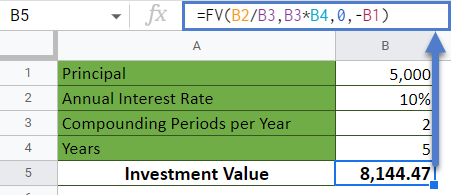# Compound Interest Formula in Excel and Google Sheets

This tutorial will demonstrate various ways to calculate compound interest in Excel and Google Sheets.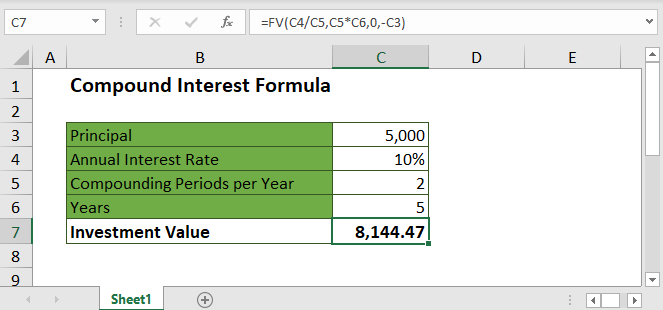## What is Compound Interest?

When you invest money, you can earn interest on your investment. Say, for example, you invest \$3,000 with a 10% annual interest rate, compounded annually. One year from the initial investment (called the principal), you earn \$300 (\$3,000 x 0.10) in interest, so your investment is worth \$3,300 (\$3,000 + \$300). For the next period, you earn interest based on the gross figure from the previous period: \$330 (\$3,300 x 0.10) because your investment was worth \$3,300. Now, it is worth \$3,630.

The general formula for compound interest is: FV = PV(1+r)n, where FV is future value, PV is present value, r is the interest rate per period, and n is the number of compounding periods.

## How to Calculate Compound Interest in Excel

One of the easiest ways is to apply the formula: (gross figure) x (1 + interest rate per period).

If you are investing \$1,000 with a 15% interest rate, compounded annually, below is how you would calculate the value of your investment after one year.

``= B2 * (1 + \$A2)``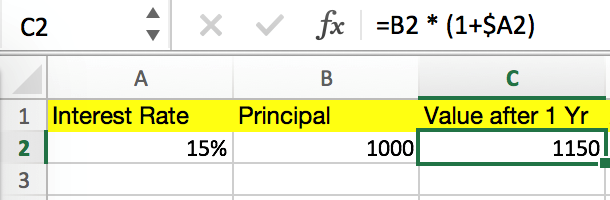In this case B2 is the Principal, and A2 is the Interest Rate per Period. The “\$” is used in the formula to fix the reference to column A, since the interest rate is constant in this example.

You can calculate the value of your investment after two years by simply copying and pasting the formula into cell D2, as shown below.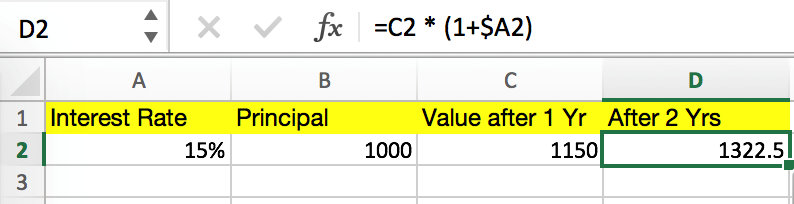C2 is the current gross figure. Notice how B2 automatically changes to C2, since the cell reference has changed. You could copy the formula over additional columns to calculate the compounded value in future years. We would recommend structuring a table like this (vertically instead of horizontally):

### Rolling Total Compound Interest Table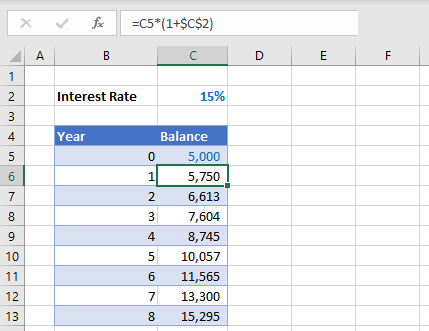The process above for calculating compound interest is easy, but if you want to figure out the value of your investment after 10 years, for example, this process is not efficient. Instead you could use a generalized compound interest formula.

## General Compound Interest Formula (for Daily, Weekly, Monthly, and Yearly Compounding)

A more efficient way of calculating compound interest in Excel is applying the general interest formula: FV = PV(1+r)n, where FV is future value, PV is present value, r is the interest rate per period, and n is the number of compounding periods.

Say, for instance that you are investing \$5,000 with a 10% annual interest rate, compounded semi-annually, and you want to figure out the value of your investment after five years. The spreadsheet below shows how this calculation can be done on Excel.

``= B1 * (1 + \$B2/2)^(B3 * B4)``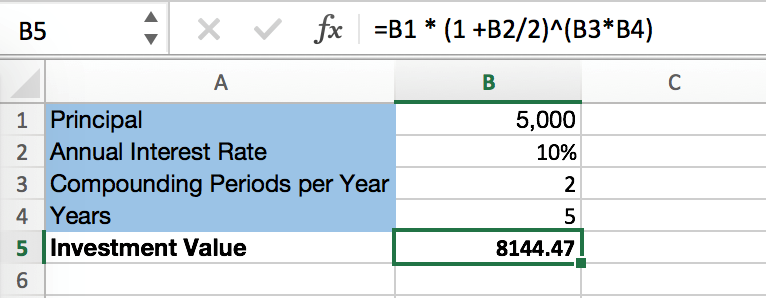In this case, PV is the Principal, r is (Annual Interest Rate) / 2 because interest is compounded semi-annually (twice per year), n is (Compounding Periods per Year) x (Year), and FV is the Investment Value. This process is more efficient than the first one because you don’t have to calculate the gross figure after each period, saving quite a few calculation steps.

## FV Function and Compound Interest

Lastly, you can calculate compound interest with Excel’s built-in Future Value Function. Similar to the previous process, the FV function calculates the future value of an investment based on the values of certain variables.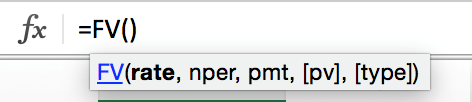The variables (as shown above) are:
rate is the interest rate for each period.

nper is the number of compounding periods.

pmt is the additional payment per period, and it is represented as a negative number. If there is no value for “pmt,” put a value of zero.

pv (optional) is the principal investment, which is also represented as a negative number. If there is no value for “pv,” you must include a value for “pmt.”

type (optional) indicates when additional payments occur. “0” indicates that the payments occur in the beginning of the period, and “1” indicates that the payments are due at the end of the period.

The previous semi-annual compound interest example can be completed with the FV function.

``= FV(B2 / B3, B3 * B4, 0, -B1)``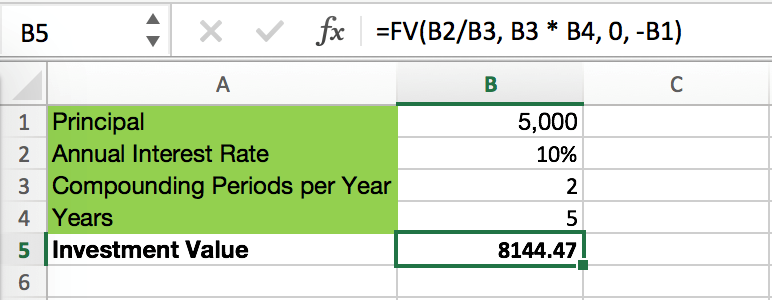The “rate” is (Annual Interest Rate) / (Compounding Period per Year), “nper” is (Compounding Periods per Year) x (Years), “pmt” is 0, and “pv” is – (Principal).

Understanding how to calculate compound interest is important for predicting investment performance, whether it’s for retirement planning or your personal portfolio, and Excel is an excellent tool to do so.